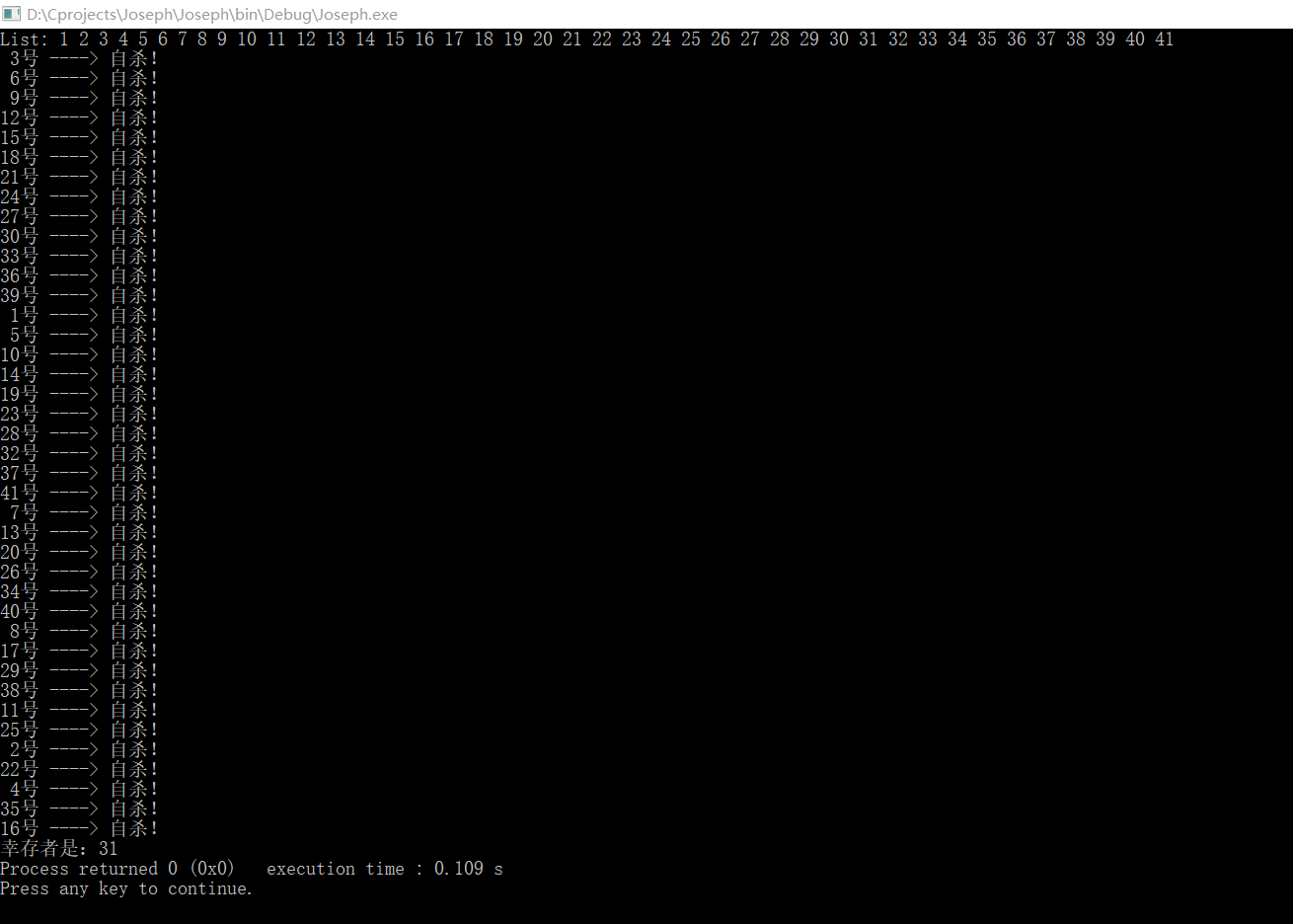## 1.首先，我们先来了解一下什么是约瑟夫环问题：

• 所有人围成一圈
• 顺时针报数，每次报到3的人将被杀掉
• 被杀掉的人将从房间内被移走
• 然后从被杀掉的下一个人重新报数，继续报3，再清除，直到剩余一人

链表的定义： 定义为编号即可 所以data项为int

``````typedef struct NODE{
struct NODE *next;
int data;
``````

``````#include <stdio.h>
#include <stdlib.h>

{
printf("List: ");
{
printf("%d ",L->data);
L = L->next;
}
printf("%d ",L->data);
printf("n");
}

int main()
{
int i;
L = (Node*)malloc(sizeof(Node));
L->data = 1;
for(i=2;i<=41;i++)
{
L->next=(Node*)malloc(sizeof(Node));
L->next->data = i;
L = L->next;
}
while(L != L->next)
{
for(i=1;i<2;i++)
{
L = L->next;
}
Out = L->next;
printf("%2d号 ----> 自杀！n",Out->data);
L ->next = Out->next;
L = L->next;
free(Out);
}
printf("幸存者是：%d",L->data);
return 0;
}
``````